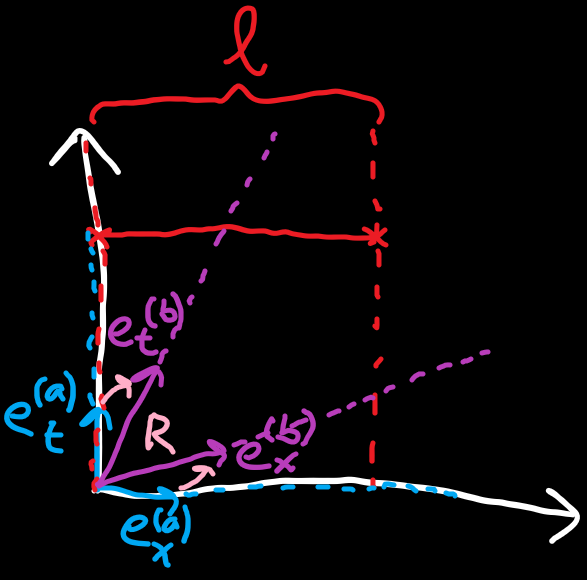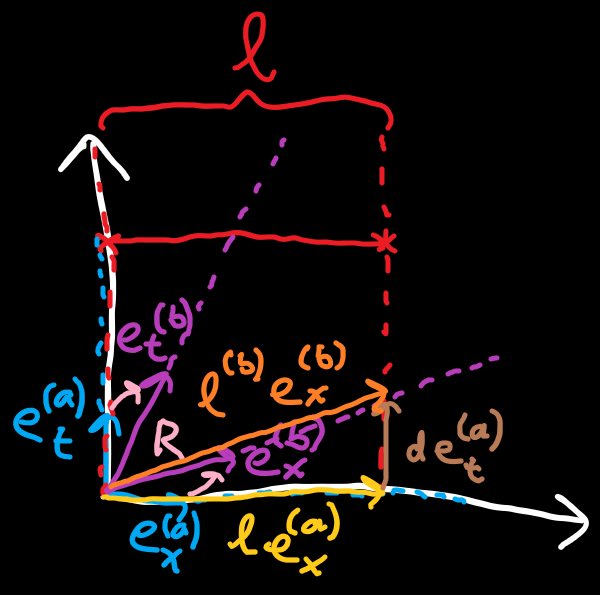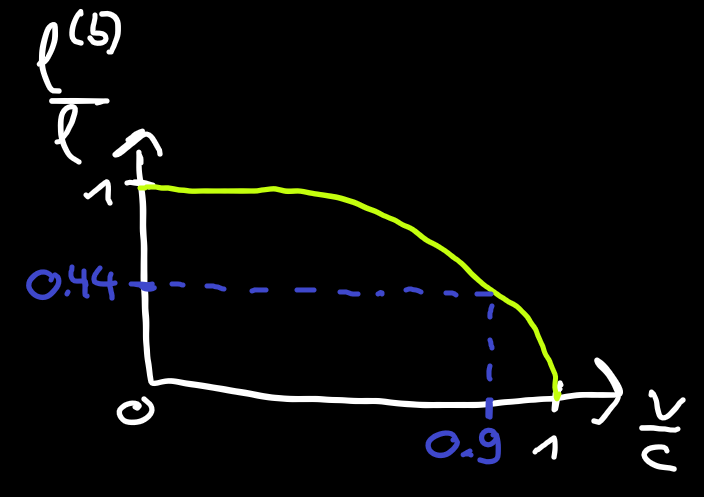### Special Relativity with Geometric Algebra - Length Contraction

In the previous section we saw how clocks tick slower for observers looking at clocks that are in motion relative to them. In this section we will look at what happens to lengths for observers moving relative to them.
This section is based on Eigenchris' video "Relativity 104c: Special Relativity - Time Dilation and Length Contraction Geometry" and the length contraction part is discussed at this part. If you have trouble following the text here it is worth watching the length contraction part of the video.

#### Length Contraction

Imagine Alice is standing still and is looking at a fixed-length object at rest with her, for example a stick. How does Alice measure the length (the x extent) of the stick? She calculates the difference vector from the first to the second endpoint and takes the inner product with her reciprocal basis vector${e}^{x}$. What is very important here: the endpoints are not separated in her time axis! She is only measuring a spatial distance and the time for both endpoints is the same.
Bob is moving relative to Alice. We have a passive transformation between Alice's and Bob's basis vectors using a rotor$R$.
The diagram below pictures our setup.Figure 2 - Blue: Alice's frame / basis vectors. Red: Stationary stick with length l. Purple: Bob's frame / basis vectors. Pink: Rotor from Alice's to Bob's frame.
So both of Bob's basis vectors are a bit tilted compared to Alice's. How does Bob see the measuring stick? If we try measuring the stick's difference vector with Bob's reciprocal basis vectors, such as in the previous section, we will run into an issue:
The stick expressed in terms of Bob's basis vectors has both a time and space component, as the stick is not parallel to either of his basis vectors. In Bob's view, the endpoints of the original stick are at different times and measuring this difference vector would not make sense as we would be measuring both a distance in space as well as a distance in time.
To remedy this we need to draw a line in Bob's frame along which events occur at the same moment in time. For that we notice that all events on lines parallel to Bob's spatial basis vector${e}_{x}^{\left(b\right)}$occur at the same time because${{e}^{t}}^{\left(b\right)}\cdot {e}_{x}^{\left(b\right)}=0$, meaning Bob's time measurement does not change along the line in Bob's x direction. They are called lines of simultaneity as events on them occur at the same time.Figure 3 - Orange: Stick along Bob's lines of simultaneity. The yellow and brown lines can be expressed in Alice's frame and relate to the orange line.
The orange vector in the drawing is a line of simultaneity because it is parallel to Bob's${e}_{x}^{\left(b\right)}$basis vector. The${e}_{x}^{\left(b\right)}$component of it in Bob's view is what we actually want to measure.
We then observe that the yellow vector with known$l$plus the brown vector with unknown$d$can be expressed with Alice's basis vectors and must equal the orange vector we're looking for which is expressed with Bob's basis vectors. So we can write
$\begin{array}{}\text{(1)}& l{e}_{x}^{\left(a\right)}+d{e}_{t}^{\left(a\right)}={l}^{\left(b\right)}{e}_{x}^{\left(b\right)}\end{array}$
We can express Bob's spatial basis vector in terms of Alice's basis vector using the rotor$R$in the opposite direction, ie.$\stackrel{~}{R}$. Doing this, expanding the rotor's exponential and simplifying yields
$\begin{array}{}\text{(2)}& \begin{array}{rl}{l}^{\left(b\right)}{e}_{x}^{\left(b\right)}& ={l}^{\left(b\right)}\stackrel{~}{R}{e}_{x}^{\left(b\right)}R={l}^{\left(b\right)}\left(\mathrm{cosh}\left(\phi \right){e}_{x}^{\left(a\right)}+\mathrm{sinh}\left(\phi \right){e}_{t}^{\left(a\right)}\right)\end{array}\end{array}$
Putting together$\text{(1)}$and$\text{(2)}$we get
$\begin{array}{}\text{(3)}& l{e}_{x}^{\left(a\right)}+d{e}_{t}^{\left(a\right)}={l}^{\left(b\right)}\left(\mathrm{cosh}\left(\phi \right){e}_{x}^{\left(a\right)}+\mathrm{sinh}\left(\phi \right){e}_{t}^{\left(a\right)}\right)\end{array}$
If we now only look at the spatial${e}_{x}^{\left(a\right)}$components of both sides we are left with
$\begin{array}{}\text{(4)}& l={l}^{\left(b\right)}\mathrm{cosh}\left(\phi \right)\end{array}$
Let's solve this for the ratio between Bob's and Alice's lengths
$\begin{array}{}\text{(5)}& \frac{{l}^{\left(b\right)}}{l}=\frac{1}{\mathrm{cosh}\left(\phi \right)}\end{array}$
Because the hyperbolic cosine function is always greater or equal to$1$, the right hand side is always less than or equal to$1$. This means that Bob, who is moving relative to the stick, always sees the length as shorter than Alice, who is at rest relative to the stick.
A observer sees lengths in relative motion shorter than lengths at rest. This is called length contraction. Let's substitute$\phi$in$\text{(5)}$with the velocity to get the relation between the velocity and the fraction of the length seen by a moving observer
$\begin{array}{}\text{(6)}& \frac{{l}^{\left(b\right)}}{l}=\frac{1}{\mathrm{cosh}\left({\mathrm{tanh}}^{-1}\left(\frac{v}{c}\right)\right)}\end{array}$
Evaluating this for$v=0.9c$we get around 44%. So an observer moving at 90% of the speed of light would see the stick at 44% of its original length at rest. Here's a plot of the relation and the value we just got.Figure 3 - Orange: Stick along Bob's lines of simultaneity. The yellow and brown lines can be expressed in Alice's frame and relate to the orange line.
The ratio starts at$1$and, as the velocity gets closer to the speed of light, the ratio shrinks to$0$. The effects start off slow and get more dramatic towards the speed of light.

#### Gamma factor

To relate with the ordinary formalism, we can also write our results in terms of the gamma factor again:
$\begin{array}{}\text{(7)}& \frac{{l}^{\prime }}{l}=\frac{1}{\mathrm{cosh}\left({\mathrm{tanh}}^{-1}\left(\frac{v}{c}\right)\right)}=\sqrt{1-\frac{{v}^{2}}{{c}^{2}}}=\frac{1}{\gamma }\end{array}$

#### Conclusion

We looked at a length in a rest frame and at how the length is seen by an observer moving relative to it. We noticed for length measurements it is important to measure both endpoints at the same time in the measurer's frame. The length ratio we arrived at varies between$1$and$0$as the relative speed goes up.

#### Formulas

• Lines of simultaneity:${e}^{t}\cdot v=0$
• Length contraction for observer moving with relative velocity$v$:$\frac{{l}^{\prime }}{l}=\frac{1}{\gamma \left(v\right)}$

#### Up next

Next we will look at how a moving observer sees an electric field in electromagnetics.